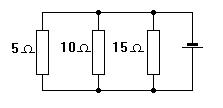Home > Electronics Tutorials > Direct Current (DC) Theory > Resistors in Parallel Tutorial

Direct Current (DC) Theory - Electronics Teaching

# Resistors in Parallel TutorialResistors in parallel are connected across one another. They all have the same voltage across them. To find the equivalent resistance (the total resistance offered to the flow of current) we invert the values and add them. Then we invert the result.

For example take 2 ohms and 4 ohms in parallel.

Inverted 1/2 +1/4 = 3/4

Invert this 4/3 = 1.33 ohms

A quick check on your answer is that it should be smaller in value than the value of the smallest resistor. If these resistors were connected across a 10 volt supply Ohms Law says about 7.5 amps would flow.

The formula can be written as 1/Rtotal = 1/R1 + 1/R2 + 1/R3 etc etc.

If only two resistors are involved then use (R1 x R2) divided by (R1 + R2)
For the 2 ohms and 4 ohms.
R1 x R2 = 8.
R1 + R2 = 6.
8/6 = 1.33 ohms

If you have several resistors of the same value in parallel then the equivalent resistance is the resistor value divided by the number of resistors.

For example, four 100 ohm resistors in parallel will provide a resistance of 25 ohms

Note: To report broken links or to submit your projects, tutorials please email to Webmaster

 Discover